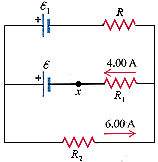# Applying Kirchoff's rule

Petey_Smalls

## Homework Statement

In the circuit shown in fig. 27-29, ε1 = 35.0, R1 = 6.00 , and R2 = 1.00 .a. Find the current in resistor R.
b. Find the resistance R
c. Find the unknown emf. ε.
d. If the circuit is broken at point x, what is the magnitude of the current in the 35.0 V battery?

sigmaI=0
sigmaV=0

## The Attempt at a Solution

I got that the current is 2 A, based on the sum of currents at a junction. But I can't solve for the resistance of R. I tried V=IR, with I=2, but I guess I was using the wrong V, since 6 is not the answer to b. Then I figured that I needed b to solve for c, so I came here looking for some help. Thanks in advance.